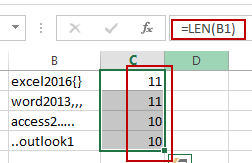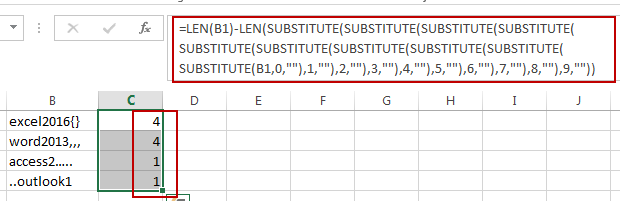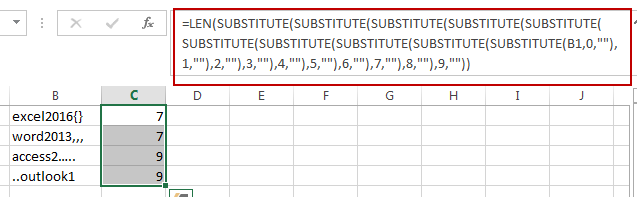# How to Count the Number of Letters or Numbers separately in a Cell in Excel

This post will guide you how to count the number of a given character in a cell in Excel. How do I count only numbers within a single cell excluding all letters and other characters with a formula in Excel. How to count the number of letters excluding all numbers in a given string in Excel.

## Count Total Characters in a Cell

Assuming that you have a list of data in range B1:B4, and you want to count the total number of all characters in one cell, you can use a formula based on the LEN function to get it. Like this:

`=LEN(B1)`

Type this formula in cell C1 and press Enter key on your keyboard, and drag the AutoFill Handle to copy this formula from Cell C1 to range C2:C4.You should notice that the number of all characters in each cell is calculated.

## Count Only Numbers in a Cell

If you want only count the total numbers in cells, excluding letters and other specific characters, you can use a formula based on the LEN function and the SUBSTITUTE function. Like this:

`=LEN(B1)-LEN(SUBSTITUTE(SUBSTITUTE(SUBSTITUTE(SUBSTITUTE(SUBSTITUTE(SUBSTITUTE(SUBSTITUTE(SUBSTITUTE(SUBSTITUTE(SUBSTITUTE(B1,0,""),1,""),2,""),3,""),4,""),5,""),6,""),7,""),8,""),9,""))`

Type this formula into a blank cell and press Enter key. And then drag the AutoFill Handle down to other cells to apply this formula.## Count Only Letters or Other Characters Excluding Numbers

If you want to count only letters and other specific characters in Cells, you can also use another formula based on the LEN function and the SUBSTITUTE function to achieve the result. Like this:

`=LEN(SUBSTITUTE(SUBSTITUTE(SUBSTITUTE(SUBSTITUTE(SUBSTITUTE(SUBSTITUTE(SUBSTITUTE(SUBSTITUTE(SUBSTITUTE(SUBSTITUTE(B1,0,""),1,""),2,""),3,""),4,""),5,""),6,""),7,""),8,""),9,""))`

Type this formula into a blank cell and press Enter key. And then drag the AutoFill Handle down to other cells to apply this formula.### Related Functions

• Excel Substitute function
The Excel SUBSTITUTE function replaces a new text string for an old text string in a text string.The syntax of the SUBSTITUTE function is as below:= SUBSTITUTE  (text, old_text, new_text,[instance_num])….
• Excel LEN function
The Excel LEN function returns the length of a text string (the number of characters in a text string).The syntax of the LEN function is as below:= LEN(text)…